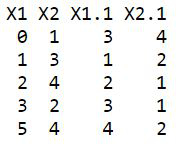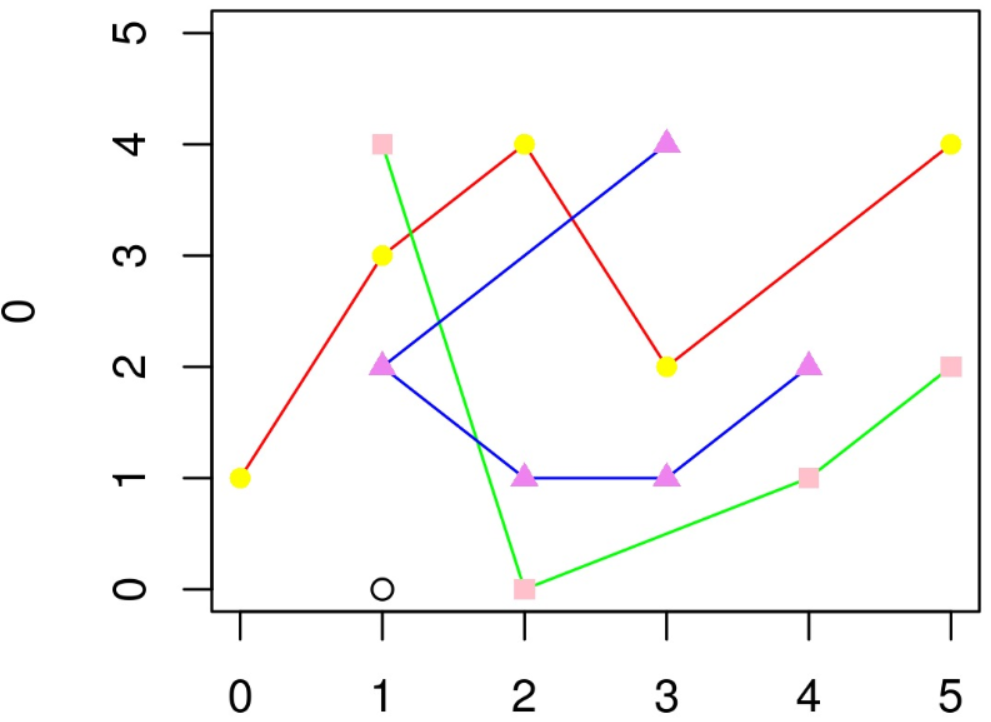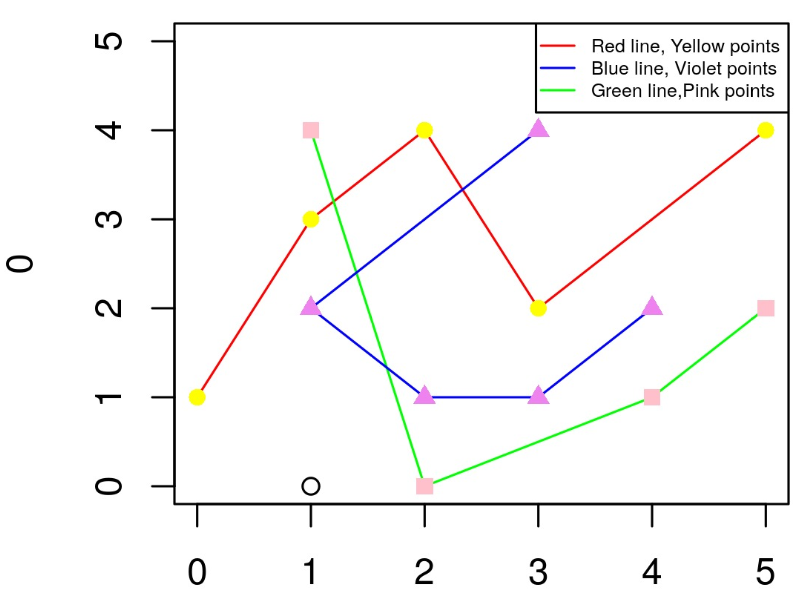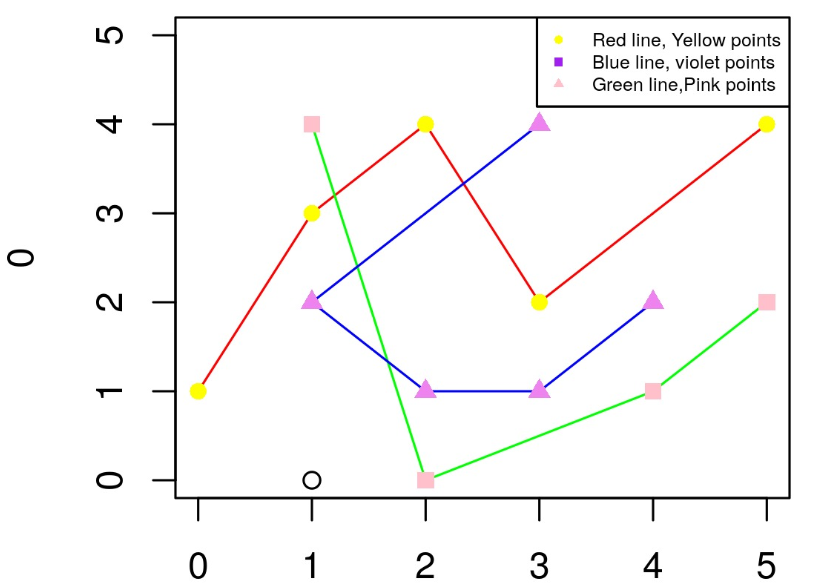Related Articles

# Different Colors of Points and Lines in Base R Plot Legend

• Difficulty Level : Basic
• Last Updated : 25 Aug, 2021

In this article, we are going to see how to plot different colors of points and lines in base R Programing Language.

Creating a dataset for demonstration:

## R

 `A <- ``matrix``( ``c``( ``c``(0,1,2,3,5), ``c``(1,3,4,2,4)), ncol=2 )``B <- ``matrix``( ``c``( ``c``(3,1,2,3,4), ``c``(4,2,1,1,2)), ncol=2 )``C <- ``matrix``( ``c``( ``c``(1,1,2,4,5), ``c``(4,4,0,1,2)), ncol=2 )``data <- ``data.frame``(A,B)``data`

Output:Let’s see in the above code where ‘A’,’B’,’C’ are the names of a matrix with vector input inside c() consisting of values. It also contains the number of rows, i.e., and ‘ncol’ as two.

### Plotting line and point on graph with different color

After creating the matrix, now we will plot the lines and points.

Syntax: plot(x, y, type = “l”, lty = 1)

lines(x, y, type = “l”, lty = 1)

Parameters:

• x, y: coordinate vectors of points to join
• type: character indicating the type of plotting. Allowed value is : “p” for points
• lty: line types. Line types can either be specified as an integer (0=blank, 1=solid (default), 2=dashed, 3=dotted, 4=dotdash, 5=longdash, 6=twodash) or as one of the character strings “blank”, “solid”, “dashed”, “dotted”, “dotdash”, “longdash”, or “twodash”, where “blank” uses ‘invisible lines’ (i.e., does not draw them).

## R

 `# Plotting the lines and points``# here type b means we are plotting``# both points and line``plot``( 0, type = ``"b"``, xlim = ``c``(0,5),``     ``ylim = ``c``(0,5) )` `# defining the line column and colour``lines``( A, col = ``"red"` `)` `# defining the point column and colour``# here pch means the type of symbol``# we can choose from 1 to 25``points``( A, col = ``"yellow"``, pch = 16 )``lines``( C, col = ``"green"` `)``points``( C, col = ``"pink"``, pch = 15 )``lines``( B, col = ``"blue"` `)``points``( B, col = ``"violet"``, pch = 17 )`

Output:In this above graph, we have lines and points plotted. There is no specification which line represents what, In order to do that we’ll the legend function to give them the same labels.

When adding a legend to a plot, there is the main way to modify the legend position.  You can set the argument x to “top”, “topleft”, “topright”, “bottom”, “bottomleft”, “bottomright”, “left”, “right” or “center”, In this scenario you don’t have to set the argument y.

In the code below, after plotting the graph we now add a legend with pch=c(NA, NA) it means that the legend section did not show the  ‘pch’ meaning the points symbol’s which we can vary from 1 to 25 as per the user’s choice.

## R

 `A <- ``matrix``( ``c``( ``c``(0,1,2,3,5), ``c``(1,3,4,2,4)), ncol=2 )``B <- ``matrix``( ``c``( ``c``(3,1,2,3,4), ``c``(4,2,1,1,2)), ncol=2 )``C <- ``matrix``( ``c``( ``c``(1,1,2,4,5), ``c``(4,4,0,1,2)), ncol=2 )``data <- ``data.frame``(A,B)` `plot``( 0, type = ``"b"``, xlim = ``c``(0,5),``     ``ylim = ``c``(0,5) )``lines``( A, col = ``"red"` `)``points``( A, col = ``"yellow"``,``       ``pch = 16 )``lines``( C, col = ``"green"` `)``points``( C, col = ``"pink"``, pch = 15 )``lines``( B, col = ``"blue"` `)``points``( B, col = ``"violet"``, pch = 17 )` `legend``( x = ``"topright"``,``        ``legend = ``c``(``"Red line, Yellow points"``,"Blue line,``                  ``iolet points``","``Green line,Pink points"),``        ``col = ``c``(``"red"``,``"blue"``,``"green"``), lwd=1, lty=``c``(1,1,1),``        ``pch=``c``(``NA``,``NA``), cex = 0.5 )`

Output:### Adding point symbols to the legend by overlapping

Now we will just overlap the legend, symbols, as we have observed previously, the lines, are visible line the legend instead of point symbols.

So what we will do, we will assign the values to “pch” which we can vary from 1 to 25 as per the user choice every number represents a point shape i.e. pch =1, which is an empty circle, pch = 19 (solid circle), pch = 21 (filled circle), etc.

## R

 `# data``A <- ``matrix``( ``c``( ``c``(0,1,2,3,5), ``c``(1,3,4,2,4)), ncol=2 )``B <- ``matrix``( ``c``( ``c``(3,1,2,3,4), ``c``(4,2,1,1,2)), ncol=2 )``C <- ``matrix``( ``c``( ``c``(1,1,2,4,5), ``c``(4,4,0,1,2)), ncol=2 )``data <- ``data.frame``(A,B)` `# plot``plot``( 0, type = ``"b"``, xlim=``c``(0,5), ylim=``c``(0,5) )``lines``( A, col = ``"red"` `)``points``( A, col = ``"yellow"``, pch=16 )``lines``( C, col = ``"green"` `)``points``( C, col = ``"pink"``, pch=15 )``lines``( B, col = ``"blue"` `)``points``( B, col = ``"violet"``, pch=17 )` `# legend``legend``( x=``"topright"``,``        ``legend=``c``(``"Red line, Yellow points"``,"Blue line,``                ``iolet points``","``Green line,Pink points"),``        ``col=``c``(``"red"``,``"blue"``,``"green"``), lwd=1, lty=``c``(1,1,1),``        ``pch=``c``(``NA``,``NA``),cex=0.5 )` `legend``( x=``"topright"``,``        ``legend=``c``(``"Red line, Yellow points"``,"Blue line,``                ``violet points``","``Green line,Pink points"),``        ``col=``c``(``"yellow"``,``"purple"``,``"pink"``), lwd=1, lty=``c``(0,0,0),``        ``pch=``c``(16,15,17),cex=0.5 )`

Output.

My Personal Notes arrow_drop_up